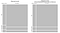# Introduction

Categorical (or discrete) variables are used to organise observations into groups that share a common trait. The trait may be nominal (e.g., sex or eye colour) or ordinal (e.g., age group, risk level), and, in general, the number of groups within a variable is 20 or fewer (Imrey & Koch, 2005).

There are various statistical procedures that can be used to analyse categorical data. For this particular piece, I’ll explain how to analyse two categorical variables by checking their statistical significance and strength of their relationship.

# Dataset

The dataset is derived from a list of sampled households. The aim of the data collection process was to track progress of drinking water, sanitation and hygiene using WHO/UNICEF Joint Monitoring Programme (JMP) service ladders.

# Data Analysis

A contingency table, also known as a cross-classification table, is a table that displays the frequency distribution of a categorical variable (s). In a contingency table, categorical variables can either be explanatory (X-Variable) or response (Y-Variable). Explanatory variables are also known as independent variables. During research they’re the ones usually manipulated to detecting changes or outcomes of the response variable.

In our household data, we’ll seek to find out if a hand washing facility can be a determinant to drinking water being contaminated. X variable will be a hand washing facility and the Y Variable will be risk level of contamination.

Using R statistical language, we’ll create a contingency table showing the distribution of the two variables.

`# Import Household Data into R Studio Workspace householdData <- read.csv(“householdData.csv”) # Data Dimension> dim(householdData)  2425 25 # The above DF has 25 columns and 2425 observations # Contingency table of presence of Hand Washing facility and risk of # E. coli contamination. handWash_RiskLevel <- table(householdData\$sdg_hand_washing, householdData\$RiskLevel, dnn = c(“HandWash”,”RiskLevel”))# Print the Contingency Table> handWash_RiskLevel                RiskLevel               HandWash       High Intermediate Low Basic/Limited  237      45       26 No facility    1781     209      127`

From the above table, we can easily check the frequency distributions of all variables. For instance, 1781 households that don’t have a hand-washing facility have high-risk levels of contamination. However, the question we need to further ask is if a hand-washing facility can affect the risk level of drinking water.

In a two-way contingency table, it’s natural to ask how X and Y variables are related. If there is no relationship between X and Y, then the categorical variables are independent i.e. probability distribution of X is not affected by presence of Y.

When performing a test for independence, we first state both the Null and the alternate hypothesis.

• Null Hypothesis: There is no relationship between the presence of a hand-washing facility and drinking water being contaminated.
• Alternate Hypothesis: There is a relationship between the presence of a hand washing facility and drinking water being contaminated.

In R, there are two ways to measure tests for independence. Fisher’s exact and Pearson’s Chi-square test for independence. For this, we’ll use the chi-square test as Fisher’s exact test is used for small sample sizes.

`chiTest <- chisq.test(handWash_RiskLevel)  > chiTest     Pearson's Chi-squared testdata:  handWash_RiskLevel X-squared = 9.9709, df = 2, p-value = 0.006837`

Looking at the results, we reject the null hypothesis and conclude that there is a relationship between the presence of a hand-washing facility and the risk of water being contaminated. This is because the p-value is lower than the significance level of 5%. To further strengthen this inference, one can plot a graph of the observed values and the expected values. Expected values are equivalent to results projected if the null hypothesis was was true.

`# Mosaic Plots of both Observed and Expected Values OP <- par(mfrow=c(1,2), "mar"=c(1,1,3,1)) mosaicplot(chiTest\$observed, cex.axis =1 , main = "Observed counts") mosaicplot(chiTest\$expected, cex.axis =1 , main = "Expected counts\n(if Hand Washing Presence had no influence)") par(OP)`Figure 1.0. Mosaic plots showing a comparison between observed and expected values.

Measures of association quantifies the relationship of X and Y variable once test for independence has been carried out. These measures can either be symmetrical or asymmetrical. Asymmetrical measures of association are performed when X variable is explanatory and Y variable is the response. Otherwise, symmetric.

Cramér’s V test is one way of measuring associations and it’s explicitly used for categorical/nominal variables. Using vcd package, I’ll measure the association between the presence of a hand-washing facility and risk level of water contamination.

`# Load the vcd package library(vcd)  # Measure the Association assocstats(handWash_RiskLevel)                     X^2   df  P(> X^2)Likelihood Ratio 9.2571  2 0.0097688 Pearson          9.9709  2 0.0068367  Phi-Coefficient   : NA  Contingency Coeff.: 0.064  Cramer's V        : 0.064`

From the code output above, Cramér’s V coefficient is very small, thus the association is weak. As an inference, presence/absence is not enough explanatory variable in predicting risk level of drinking water.

An Analytics and Business Intelligence Company

## More from Polar Analytics

An Analytics and Business Intelligence Company